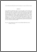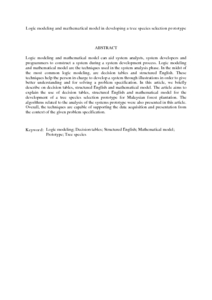# Logic modeling and mathematical model in developing a tree species selection prototype

## Citation

Kamarudin, Shafinaz and Sahari, Noraidah and Selamat, Mohd Hasan and Abdullah, Rusli and Sulaiman, Riza and Mohammad, Mohd Ikram (2014) Logic modeling and mathematical model in developing a tree species selection prototype. Journal of Theoretical and Applied Information Technology, 60 (2). pp. 331-342. ISSN 1992-8645; ESSN: 1817-3195

## Abstract

Logic modeling and mathematical model can aid system analysts, system developers and programmers to construct a system during a system development process. Logic modeling and mathematical model are the techniques used in the system analysis phase. In the midst of the most common logic modeling, are decision tables and structured English. These techniques help the person in charge to develop a system through illustrations in order to give better understanding and for solving a problem specification. In this article, we briefly describe on decision tables, structured English and mathematical model. The article aims to explain the use of decision tables, structured English and mathematical model for the development of a tree species selection prototype for Malaysian forest plantation. The algorithms related to the analysis of the systems prototype were also presented in this article. Overall, the techniques are capable of supporting the data acquisition and presentation from the context of the given problem specification.Preview
PDF (Abstract)
Logic modeling and mathematical model in developing a tree species selection prototype.pdfView Item